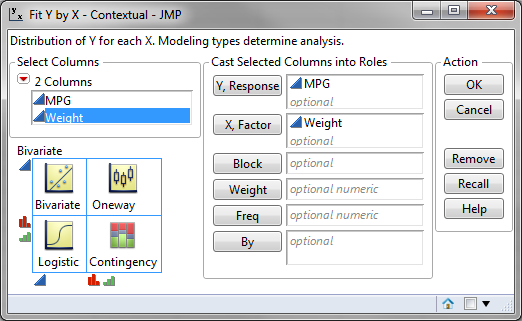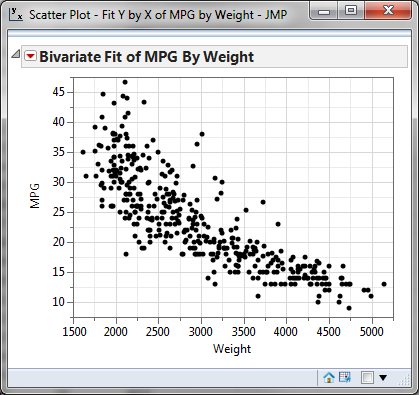# Scatter Plot using JMP

### What is a Scatter Plot?

A scatter plot is a diagram to present the relationship between two data set variables. A scatterplot consists of a set of data points. On the scatterplot, a single observation is presented by a data point with its horizontal position equal to the value of one variable and its vertical position equal to the value of the other variable. A scatterplot helps us to understand:

• Whether the two variables are related to each other or not
• What is the strength of their relationship
• The shape of their relationship
• The direction of their relationship
• Whether outliers are present

### How to Use JMP to Generate a Scatter Plot

Data File: “ScatterPlot.jmp”

#### Steps to render a scatter plot in JMP:

1. Click Analyze -> Fit Y by X
2. Select MPG as the “Y, Response” and weight as the “X, Factor”3. Click OKModel summary: The figure above is JMP’s output of the scatterplot data. You can immediately see the value of graphical displays of data. This information obtainable by viewing this output shows a relationship between weight and MPG. This scatterplot shows that the heavier the weight, the lower the MPG value and vice versa.